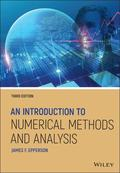John Wiley & Sons An Introduction to Numerical Methods and AnalysisThe new edition of the popular introductory textbook on numerical approximation methods and mathemat.. Product #: 978-1-119-60469-3 Regular price: \$116.82 \$116.82 Auf Lager

# An Introduction to Numerical Methods and Analysis3. Auflage Oktober 2021
672 Seiten, Hardcover
Fachbuch

ISBN: 978-1-119-60469-3
John Wiley & Sons

Jetzt kaufen

### Preis: 125,00 €

ca.-Preis

Preis inkl. MwSt, zzgl. Versand

The new edition of the popular introductory textbook on numerical approximation methods and mathematical analysis, with a unique emphasis on real-world application

An Introduction to Numerical Methods and Analysis helps students gain a solid understanding of a wide range of numerical approximation methods for solving problems of mathematical analysis. Designed for entry-level courses on the subject, this popular textbook maximizes teaching flexibility by first covering basic topics before gradually moving to more advanced material in each chapter and section. Throughout the text, students are provided clear and accessible guidance on a wide range of numerical methods and analysis techniques, including root-finding, numerical integration, interpolation, solution of systems of equations, and many others.

This fully revised third edition contains new sections on higher-order difference methods, the bisection and inertia method for computing eigenvalues of a symmetric matrix, a completely re-written section on different methods for Poisson equations, and spectral methods for higher-dimensional problems. New problem sets--ranging in difficulty from simple computations to challenging derivations and proofs--are complemented by computer programming exercises, illustrative examples, and sample code. This acclaimed textbook:
* Explains how to both construct and evaluate approximations for accuracy and performance
* Covers both elementary concepts and tools and higher-level methods and solutions
* Features new and updated material reflecting new trends and applications in the field
* Contains an introduction to key concepts, a calculus review, an updated primer on computer arithmetic, a brief history of scientific computing, a survey of computer languages and software, and a revised literature review
* Includes an appendix of proofs of selected theorems and a companion website with additional exercises, application models, and supplemental resources

An Introduction to Numerical Methods and Analysis, Third Edition is the perfect textbook for upper-level undergraduate students in mathematics, science, and engineering courses, as well as for courses in the social sciences, medicine, and business with numerical methods and analysis components.

JAMES F. EPPERSON, PHD, has been Associate Editor of Mathematical Reviews, published by the American Mathematical Society, since 2001, and will be retiring from this position in July 2021. He was previously Associate Professor in the Department of Mathematical Sciences at the University of Alabama in Huntsville. Dr. Epperson is the author of the previous editions of An Introduction to Numerical Methods and Analysis and their accompanying solutions manuals.

J. F. Epperson, University of Alabama in Huntsville